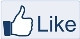# IMAGE PROCESSING

" Two roads diverged in a wood, and I,
I took the one less traveled by,
And that has made all the difference "-Robert Frost

### Digital Image Water Marking – Part 1

Digital image watermarking is the method in which data is embedded in a multimedia file such as an image or a video, so as to verify the credibility of the content or the identity of the owner.

MATLAB CODE:
%Digital Image Watermarking

%Watermark
figure,imshow(w);title('Watermark');

%Visibility of the watermark
alpha=0.5;

Fw = (1-alpha)*f + alpha.*w;
figure,imshow(Fw);title('Watermarked Image-Visibilty:0.5');

Explanation:
1.      Read the image to be watermarked. In my case it ‘s ‘angel.jpg’
2.      Read the watermark. Make sure the image size and the watermark size are same.
3.      Adjust the alpha value based on your requirement. Alpha can be between 0 and 1. If alpha is less than 0.5 the watermark will be less visible and if the alpha is more than 0.5, the watermark will be clearly visible.

MATLAB CODE:

figure,imshow(Logo);title('Blog Logo');

OIm = f;
%Visibility control
alpha = 0.5;

[m, n,~]=size(Logo);

%choose a portion of the Image
Sz = [495 927];

%Apply watermark
OIm(Sz(1):Sz(1)+m-1,Sz(2):Sz(2)+n-1,:)=(1-alpha)*OIm(Sz(1):Sz(1)+m-1,Sz(2):Sz(2)+n-1,:) + alpha.*Logo;
figure,imshow(OIm);title('Watermark in the centre');

EXPLANATION:
2.      Note: The size of the input image and the logo are not same.
3.      Choose a portion in the input image and apply watermark.

LSB Digital Watermarking
In this method, the watermark is hidden in the input image.
Consider a pixel from the input image; say 249 and a pixel from the watermark say, 155.
Now hide the pixel value of the watermark in the two least significant bits of the input image.
1.      The binary representation of 249 is 11111001
2.      The binary representation of 155 is 10011011
3.      Make the two least significant bits of input image value 249 to zeros i.e. 11111000
4.      Find the two Most significant bits of the watermark value 155 i.e. 10
5.      Now place the two most significant bits of the watermark at the two least significant bits of the input image.
6.      11111000 + 00000010 = 11111010 i.e.  250

MATLAB CODE:
%LSB watermarked Image
Fw1 = 4*(f/4) + w/64;
figure,imshow(Fw1);title('LSB watermarked Image');

How to retrieve the watermark:
Method 1:
Subtract the input image (without watermark) from the watermarked Image.
%Retrieve the Watermark
DMark = (Fw1-f)*64;
figure,imshow(DMark);title('Watermark extracted from the image');

Method 2:
Retrieve the two least significant bits of the image
%Retrieve the Watermark
DMark2 = rem(Fw1,4)*64;
figure,imshow(DMark2);title('Watermark extracted from the image');

We can extend the above techniques for steganography or image coding. In the next upcoming post I will share about how to validate the digital watermark.Like "IMAGE PROCESSING" page